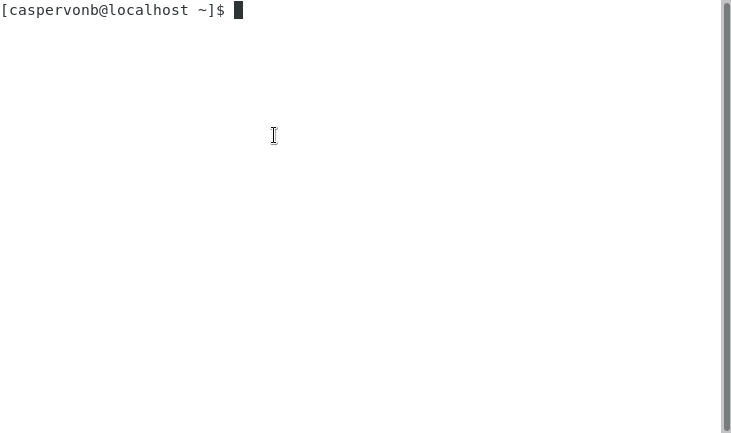# 鲜为人知的 JavaScript 陷阱

2收藏

``````const numbers = [1, 2, 3, 4];
numbers.map(function(n) {
return n * n;
});
``````

``````const numbers = [1, 2, 3, 4];
numbers.map(n => n * n);
``````

``````const numbers = [1, 2, 3, 4];
numbers.map(n => { value: n });
``````

``````const numbers = [1, 2, 3, 4];
numbers.map(function(n) {
value:
n
return;
});
``````

``````const numbers = [1, 2, 3, 4];
numbers.map(n => ({ value: n }));
``````

``````let calculator = {
value: 0,
this.value = values.reduce((a, v) => a + v, this.value);
},
};
console.log(calculator.value);
``````

``````let calculator = {
value: 0,
this.value = values.reduce((a, v) => a + v, this.value);
},
};
console.log(calculator.value);
``````

``````const adder = {
this.value = values.reduce((a, v) => a + v, this.value);
},
};
let calculator = {
value: 0
};
``````

``````return
{
value: 42
}
``````

``````return;
{
value: 42
};
``````

``````let set = new Set();
console.log(set.length);
``````

``````let set = new Set();
console.log(set.size);
``````

``````let segment = new Segment();
function Segment() {
this.x = 0;
this.y = 0;
}
``````

``````let segment = new Segment();
class Segment {
constructor() {
this.x = 0;
this.y = 0;
}
}
``````

Finally

Finally 是一个特殊情况。看一下以下代码片段：

``````try {
return true;
} finally {
return false;
}
``````

JavaScript 很容易入门，但很难精通。换句话说，开发人员需要搞清楚自己正在做什么，明白为什么要这样做，否则就很容易出错。

ECMAScript 6 及其含糖功能尤其如此。特别是箭头函数哪里都会冒出来。让我来猜的话，那是因为开发人员认为它们比常规函数更漂亮。但它们不是常规函数，因此无法替代后者。

AST Explorer 之类的工具还可以帮助你了解某些极端场景下的状况。人类和计算机往往会以不同的方式来解析事物。1

1条回复

/

2020-9-8 16:19:56帖子
视频
声望
粉丝
社区精华内容